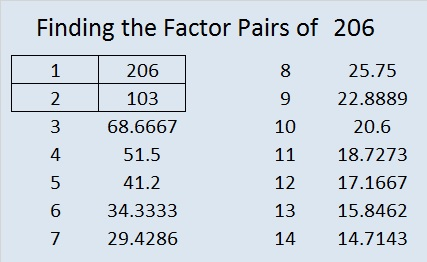# 206 and Level 6

• 206 is a composite number.
• Prime factorization: 206 = 2 x 103
• The exponents in the prime factorization are 1 and 1. Adding one to each and multiplying we get (1 + 1)(1 + 1) = 2 x 2 = 4. Therefore 206 has exactly 4 factors.
• Factors of 206: 1, 2, 103, 206
• Factor pairs: 206 = 1 x 206 or 2 x 103
• 206 has no square factors that allow its square root to be simplified. √206 ≈ 14.35270009——————————————————————

Excel file of puzzles and previous week’s factor solutions: 12 Factors 2014-08-11This site uses Akismet to reduce spam. Learn how your comment data is processed.